# ggside walkthrough

``````library(dplyr)
#>
#> Attaching package: 'dplyr'
#> The following objects are masked from 'package:stats':
#>
#>     filter, lag
#> The following objects are masked from 'package:base':
#>
#>     intersect, setdiff, setequal, union
library(ggplot2)
library(ggside)``````

# ggside

The package ggside was designed to enable users to add metadata to their ggplots with ease. While adding metadata information is not extremely difficult to do with `geom_tile` or other geoms, it can be frustrating to the user positioning these geometries away from the main plot. Additionally, if the user wants to use a color guide with the `fill` aesthetic, then they may run into conflicts when one layer uses a discrete scale and another uses a continuous scale.

# Motivation

Lets look at a very simple example set using `dplyr` to summarise the `diamonds` dataset.

``````summariseDiamond <- diamonds %>%
mutate(`Cut Clarity` = paste(cut, clarity)) %>%
group_by(`Cut Clarity`,cut, clarity, color) %>%
summarise(n = n(),
`mean Price` = mean(price),
sd = sd(price))
#> `summarise()` has grouped output by 'Cut Clarity', 'cut', 'clarity'. You can override using the `.groups` argument.
ggplot(summariseDiamond, aes(x = color, y = `Cut Clarity`)) +
geom_tile(aes(fill = `mean Price`))``````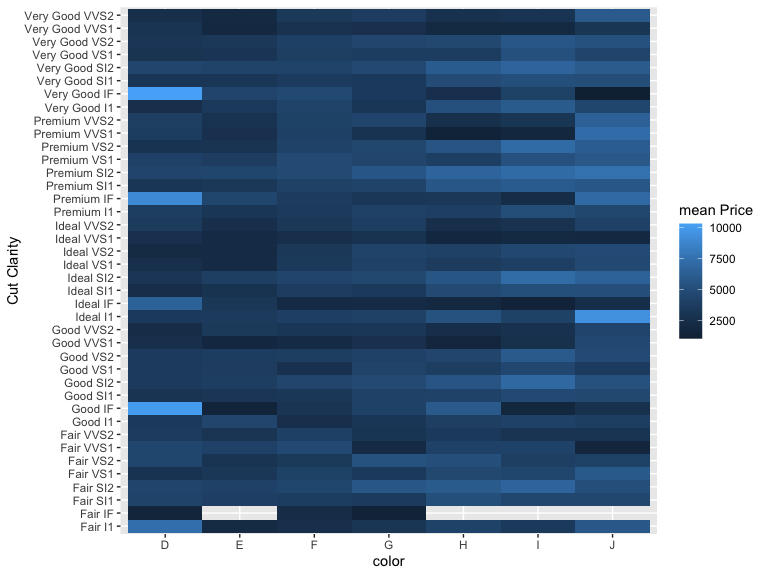``````p <-ggplot(summariseDiamond, aes(x = color, y = `Cut Clarity`)) +
geom_tile(aes(fill = `mean Price`)) +
geom_tile(aes(x=0, fill = cut))

p``````

As you can see, trying to place a colorbar causes an error because the previous `geom_tile` call has already mapped `mean Price` to `fill` and has deemed the scale as continuous. Thus a categorical variable is unable to map to the `fill` aesthetic anymore.

However, you could map another continuous variable, but this will place these to the same guide, shifting the limits and washing out all color.

``````summariseDiamond <- summariseDiamond %>%
group_by(`Cut Clarity`) %>%
mutate(`sd of means` = sd(`mean Price`))

ggplot(summariseDiamond, aes(x = color, y = `Cut Clarity`)) +
geom_tile(aes(fill = `mean Price`)) +
geom_tile(aes(x=0, fill = `sd of means`))``````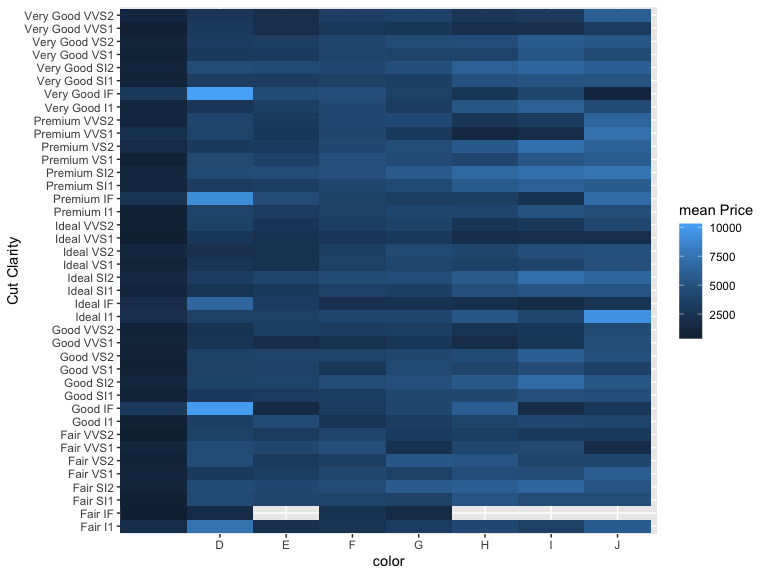Using ggside allows for aesthetics to be mapped to a separate scale, which can also be controlled with `scale_*fill_gradient` functions (more on this later).

``````ggplot(summariseDiamond, aes(x = color, y = `Cut Clarity`)) +
geom_tile(aes(fill = `mean Price`)) +
geom_ysidetile(aes(x = "sd of means", yfill = `sd of means`))  +
scale_yfill_gradient(low ="#FFFFFF", high = "#0000FF") ``````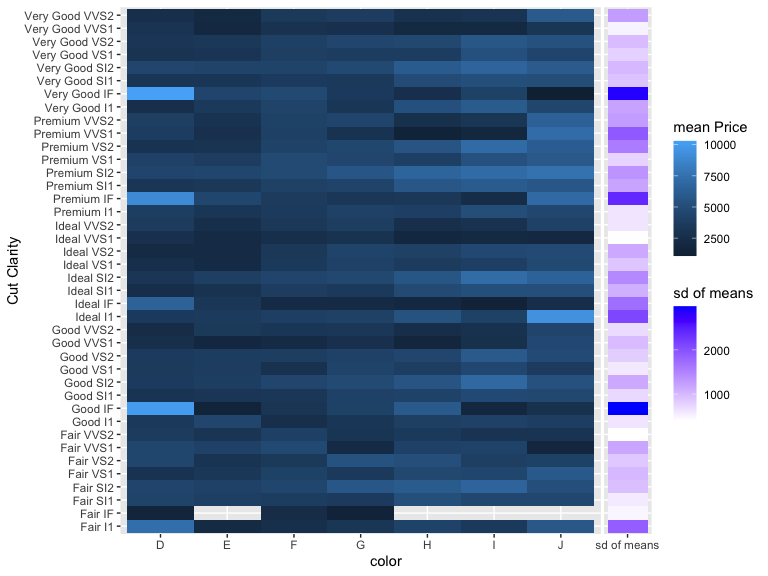``````ggplot(summariseDiamond, aes(x = color, y = `Cut Clarity`)) +
geom_tile(aes(fill = `mean Price`)) +
geom_ysidetile(aes(x = "max", yfill = after_stat(summarise),
domain = `mean Price`), stat = "summarise", fun = max) +
geom_ysidetile(aes(x = "mean",yfill = after_stat(summarise),
domain = `mean Price`), stat = "summarise", fun = mean) +
geom_ysidetile(aes(x = "median",yfill = after_stat(summarise),
domain = `mean Price`), stat = "summarise", fun = median) +
geom_ysidetile(aes(x = "min",yfill = after_stat(summarise),
domain = `mean Price`), stat = "summarise", fun = min) +
scale_yfill_gradient(low ="#FFFFFF", high = "#0000FF") ``````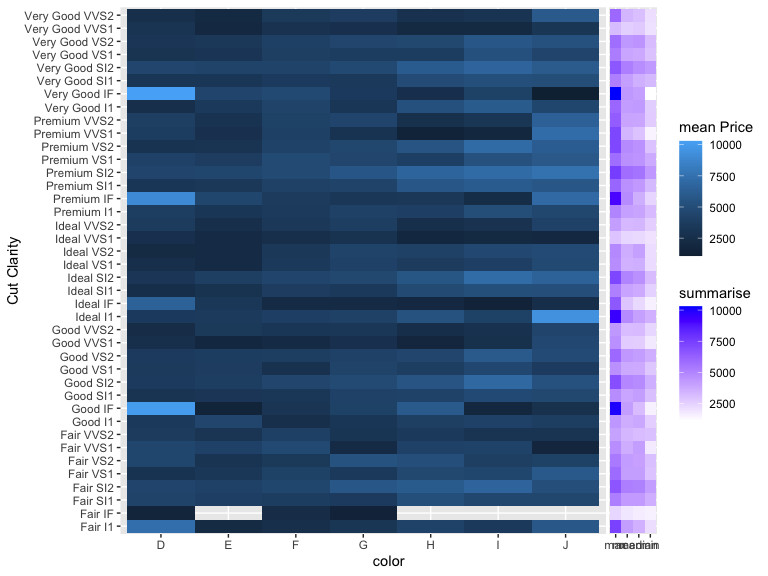``````.tmp <- summariseDiamond %>% group_by(`Cut Clarity`) %>%
summarise_at(vars(`mean Price`),.funs = list(max,median,mean,min)) %>%
tidyr::gather(key = key, value = value, -`Cut Clarity`)

ggplot(summariseDiamond, aes(x = color, y = `Cut Clarity`)) +
geom_tile(aes(fill = `mean Price`)) +
geom_ysidetile(data = .tmp, aes(x = key, yfill = value)) +
scale_yfill_gradient(low ="#FFFFFF", high = "#0000FF") ``````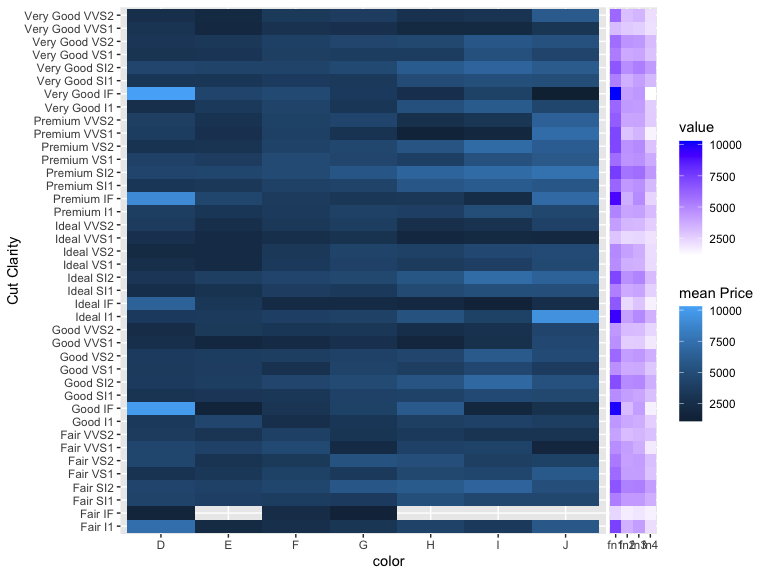Unfortunately using `xfill` or `yfill` with `geom_xsidetile` or `geom_ysidetile` respectively will lock its associated scale with the first layer. So you cannot first assign `yfill` to a discrete scale and then add a layer with `yfill` maps to a continuous variable or vise a versa. For example, the following code still produces an error. This is largely due to the original motivation for making this package, but at least ggside can give some ease to plotting information to the sides of the main figure.

``````p <- ggplot(summariseDiamond, aes(x = color, y = `Cut Clarity`)) +
geom_tile(aes(fill = `mean Price`)) +
geom_ysidetile(aes(yfill = `sd of means`)) + #sets yfill to a continuous scale
geom_ysidetile(aes(yfill = cut)) #attempting to add discrete color values

p``````

# How to Use

## Geom

`geom_xside*` and `geom_yside*` both extend the `ggplot2::Geom*` environments. As you may expect, `geom_xside*` allows you to place geometries along the x-axis and `geom_yside*` allows placement along the y-axis. All of the `geom_*side*` functions provide a variation on the color aesthetics `colour`/`fill`. The variants are named `xcolour` and `xfill` or `ycolour` and `yfill` for their respective `xside` or `yside` geoms. These aesthetics will take precedence over their more general counterpart if assigned. This allows for certain geoms to be plotted on different color scales - particularly useful when one requires a discrete scale and another requires a discrete scale.

### Available Geoms

The following geoms are currently available to use right away from the `ggside` package. Each of the following ggproto `Geom*`’s are total clones to `GeomXside*` or `GeomYside*` with the only variations being the additional color aesthetics. The `geom_*side*` functions return a ggside_layer object. When a ggside_layer is added to a ggplot, the plot is transformed into a ggside object which has a different `ggplot_build` S3 method. This method is what allows for the side geoms to be plotted on a separate panel.

• GeomBar
• GeomBoxplot
• GeomDensity
• GeomFreqpoly
• GeomHistogram
• GeomLine
• GeomPath
• GeomPoint
• GeomText
• GeomTile
• GeomViolin

## Facets

Technically speaking `ggside`’s main workhorse is hacking `Facet` framework. Whenever a standard `ggplot` object is converted to a `ggside` object, the current `Facet ggproto` class is replaced to one that is compatible with `ggside`. All `geom*side` variants are plotted in a panel adjacent to the axis their name implies. All vanilla `ggplot2` geometries are plotted in the main panel.

### How its done

#### XLayer and YLayer

Each `geom_*side*` variants function return an `XLayer` or `YLayer` which both extends `ggplot2:::Layer`. Currently, only `Layer\$setup_layer` is overwritten to add column `PANEL_TYPE` to the data. This column will contain `"x"`, or `"y"` which will help map data to the correct panel. Data missing the `PANEL_TYPE` column (or containing values other than `"x"` or `"y"`) is assumed to be mapped to the main panel. The values in `PANEL_TYPE` help predict which extra panels needed to be drawn per main panel produced by the original `Facet` class the ggplot holds.

#### Facet

Three main methods are overwritten in order to make `ggside` work. `compute_layout`, `map_data`, and `draw_panels`. The `compute_layout` will first call the base Facet’s method, and then will will build more panels based on the attached `ggside` object. `map_data` will take extra care to ensure data is mapped to the proper panel using `PANEL_TYPE` as well as any other facet variables passed. `draw_panels` which is responsible for rendering all panels correctly.

#### Extending

Currently, `ggside` works with `ggplot2`’s three base facet classes, `FacetNull`, `FacetWrap` and `FacetGrid`. If you wish to extend `ggside` to another package’s custom facet function, then you must also export a `as_ggsideFacet` S3 method, which will be called when an the `ggplot` is converted to `ggside` or whenever a new facet is added to the plot. This method should return a ggproto object that inherits from the `Facet` group. Helpful computed variables in the `layout` object are `PANEL_TYPE` which indicates if the `PANEL` expects a side geom or default geom, and `PANEL_GROUP` which helps clarify which `PANEL`’s are grouped together in a facet. These additional computed variables and the `ggside` object passed to `params` will have the information needed to help you draw panels for you custom facet with `ggside`.

### Examples with Facets

``````i2 <- iris %>%
mutate(Species2 = rep(c("A","B"), 75))
p <- ggplot(i2, aes(Sepal.Width, Sepal.Length, color = Species)) +
geom_point()``````
``````p2 <- p + geom_xsidedensity(aes(y=stat(density))) +
geom_ysidedensity(aes(x=stat(density))) +
theme_bw()
p2 + labs(title = "FacetNull")``````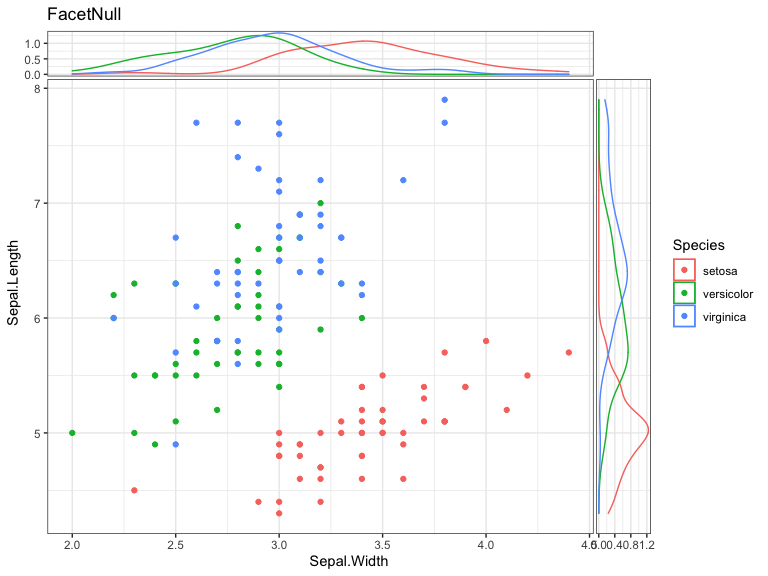``````p2 + facet_wrap(Species~Species2) +
labs(title = "FacetWrap") +
guides(guide_axis(check.overlap = T))``````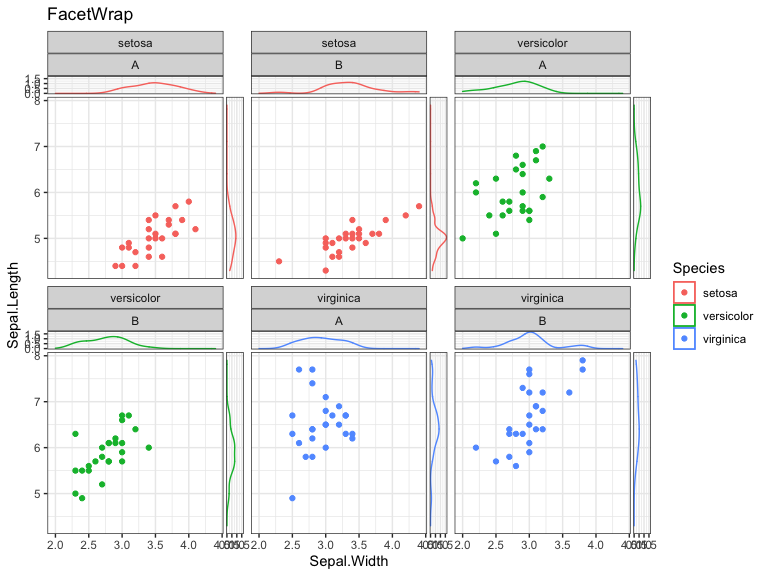``p2 + facet_grid(Species~Species2, space = "free", scale = "free_y") ``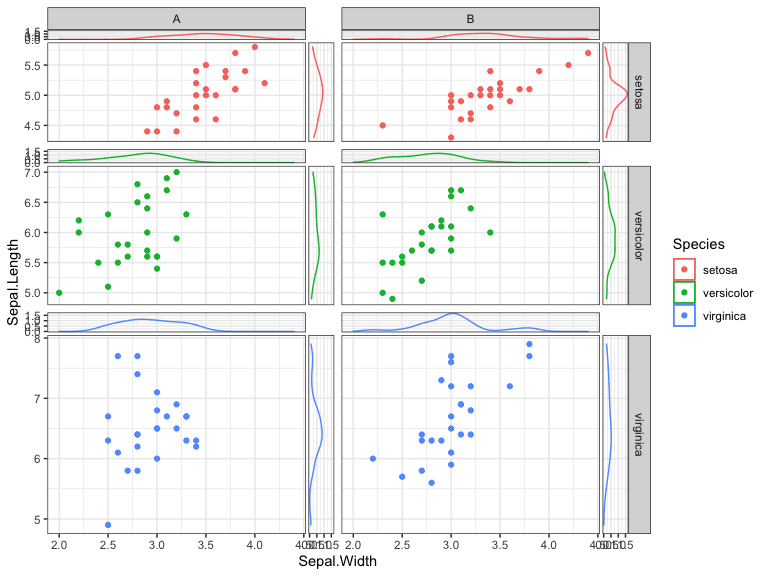Further control on how the `sideFacets` are handled may be done with the `ggside` function.

``````p2 + ggside(x.pos = "bottom", y.pos = "left") +
labs(title = "FacetNull", subtitle = "Xside placed bottom, Yside placed left")``````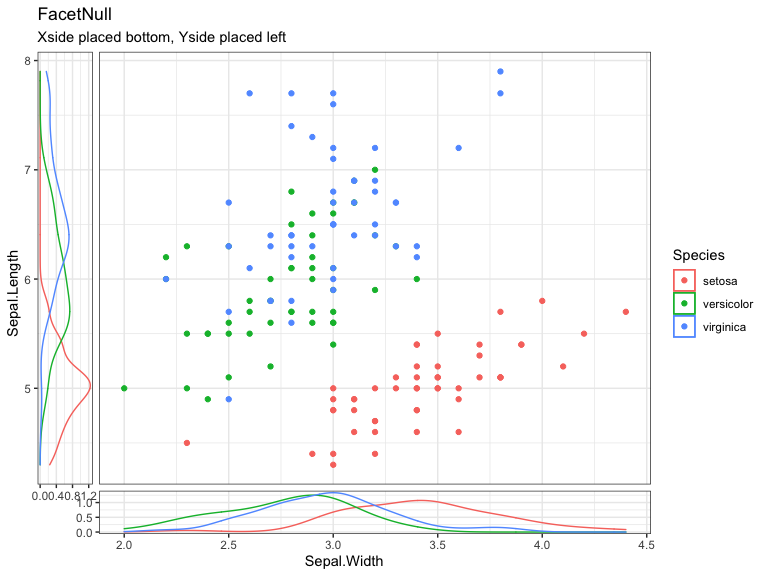When using having multiple panels, it may be handy to collapse side panels to one side, which helps save space and computation time!

``````p2 + facet_wrap(Species~Species2) +
labs(title = "FacetWrap", subtitle = "Collapsing X side Panels") +
ggside(collapse = "x") ``````